## Example Questions

← Previous 1

### Example Question #1 : X And Y Intercept

Find the slope of the following line: 6x – 4= 10

1.5

–5/2

–1.5

5/2

1.5

Explanation:

Putting the equation in y = mx + b form we obtain y = 1.5x – 2.5.

The slope is 1.5.

### Example Question #2 : X And Y Intercept

What is the x-intercept of the line in the standardcoordinate plane for the following equation?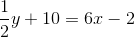2

12

3

-242

Explanation:

This question is asking us to find the x-intercept. Remember that the y-value is equal to zero at the x-intercept. Substitute zero in for the y-variable in the equation and solve for the x-variable.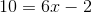Add 2 to both sides of the equation.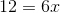Divide both sides of the equation by 6.The line crosses the x-axis at 2.

### Example Question #3 : X And Y Intercept

What is the equation of a line that has an x-intercept of 4 and a y-intercept of -6?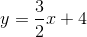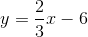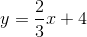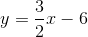Explanation:

The equation of a line can be written in the following form:In this formula, is the slope, and b represents the y-intercept. The problem provides the y-intercept; therefore, we know the following information: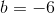We can calculate the slope of the line, because if any two points on the function are known, then the slope can be calculated. Generically, the slope of a line is defined as the function's rise over run, or more technically, the changes in the y-values over the changes in the x-values.  It is formally written as the following equation:The problem provides the two intercepts of the line, which can be written as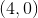and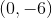. Substitute these points into the equation for slope and solve:.

Substitute the calculated values into the general equation of a line to get the correct answer:.

### Example Question #1 : How To Find X Or Y Intercept

What are the y and x intercepts of the given equation, respectively?

y = 2x – 2

(0, 2), (2, 0)

(0, –2), (1, 0)

(0, 0), (0, 0)

(0, –2), (2, 0)

(0, –2), (–2, 0)

(0, –2), (1, 0)

Explanation:

The equation is already in slope-intercept form. The y-intercept is (0, –2). Plug in 0 for y and we get the x intercept of (1, 0)

### Example Question #2 : How To Find X Or Y Intercept

What is the x-intercept of the following line?

y = –3x + 12

2

–1/4

–4

1/4

4

4

Explanation:

The x-intercept occurs when the y-coordinate = 0.

y = –3x + 12

0 = –3x + 12

3x = 12

x = 12/3 = 4

### Example Question #3 : How To Find X Or Y Intercept

What is the-coordinate of the point in the standard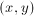coordinate plane at which the two lines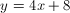and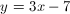intersect?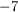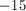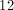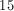Explanation: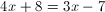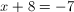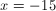### Example Question #4 : How To Find X Or Y Intercept

What is the-intercept of the line in the standard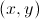coordinate plane that goes through the points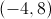and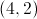?Explanation:

The answer is.

The slope of the line is determined by calculating the change inover the change in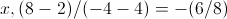.

The point-slope form of the equation for the line is then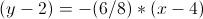. The-intercept is determined by setting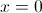and solving for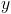. This simplifies to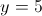which shows thatis the-interecept.

### Example Question #5 : How To Find X Or Y Intercept

What are theand-intercepts of the line defined by the equation: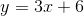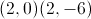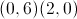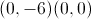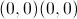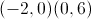Explanation:

To find the intercepts of a line, we must set theandvalues equal to zero and then solve.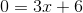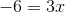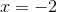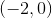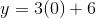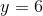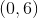### Example Question #6 : How To Find X Or Y Intercept

In the standard (x, y) coordinate plane, a circle has the equation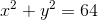. At what points does the circle intersect the x-axis?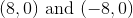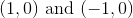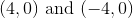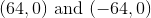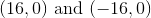Explanation:

The generic equation of a circle is (x - x0)2 + (y - y0)2 = r2, where (x0, y0) are the coordinates of the center and r is the radius.In this case, the circle is centered at the origin with a radius of 8. Therefore the circle hits all points that are a distance of 8 from the origin, which results in coordinates of (8,0) and (-8,0) on the x-axis.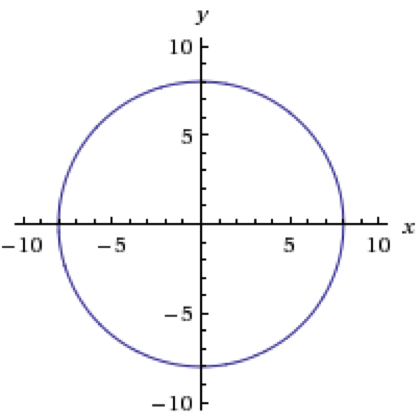### Example Question #7 : How To Find X Or Y Intercept

What is the y-intercept of a line that passes through the point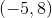with slope of?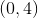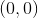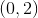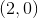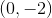Explanation:

Point-slope form follows the format y - y1 = m(x - x1).

Using the given point and slope, we can use this formula to get the equation y - 8 = -2(x + 5).

From here, we can find the y-intercept by setting x equal to zero and solving.

y - 8 = -2(0 + 5)

y - 8 = -2(5) = -10

y = -2

Our y-intercept will be (0,-2).

← Previous 1

### All ACT Math Resources